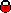## Measurement of the longitudinal structure function F_L at high Q2 at HERA

 Reference Preliminary for DIS 2008, March 2008 Document Conference paper or talk Figures (2)  (3)  (4)  (5)  (6)  (7)  (8)  (9)  (10)  (11)  (12)  (13)  (14)  (15)  (16)  (17)  (18)  (19)  (20)  (21)  (22)  (23)  (24)  (25)  (26) Contact V. Chekelian, A. Dubak, A. Nikiforov, S. Habib, A. Raspiareza, S. Shushkevich Analysis webpage Abstract A measurement of the longitudinal proton structure function $F_{L}(x,Q^{2})$ derived from inclusive deep inelastic $ep$ scattering cross section measurements at high $Q^{2}$ with the H1 detector at HERA is presented. The data were taken in the year 2007 at a positron beam energy of $E_{e}=27.5$~GeV and proton beam energies $E_{p}$ of 920~GeV, 575~GeV and 460~GeV. The measurements of the NC cross sections from this analysis combined with the reported earlier NC cross section measurements for the same data periods at medium $Q^{2}$ allowed to measure $F_{L}(x,Q^{2})$ in a range of high four-momentum transfer squared $12 \leq Q^2 \leq 800~$GeV$^2$ and correspondingly Bjorken-$x$ between $0.00028\leq x \leq 0.0353$. The measured longitudinal structure function is found to be consistent with the NLO QCD calculations. Links back to overview listingH1 internal information